Courses

# Modelling of Control Systems - 2

## 15 Questions MCQ Test Topicwise Question Bank for Electrical Engineering | Modelling of Control Systems - 2

Description
This mock test of Modelling of Control Systems - 2 for Electrical Engineering (EE) helps you for every Electrical Engineering (EE) entrance exam. This contains 15 Multiple Choice Questions for Electrical Engineering (EE) Modelling of Control Systems - 2 (mcq) to study with solutions a complete question bank. The solved questions answers in this Modelling of Control Systems - 2 quiz give you a good mix of easy questions and tough questions. Electrical Engineering (EE) students definitely take this Modelling of Control Systems - 2 exercise for a better result in the exam. You can find other Modelling of Control Systems - 2 extra questions, long questions & short questions for Electrical Engineering (EE) on EduRev as well by searching above.
QUESTION: 1

Solution:
QUESTION: 2

Solution:
QUESTION: 3

### Match List - I (Control System Components) with List - II (Applications) and select the correct answer using the codes given below the lists: List - I A. Stepper Motors B. Synchros C. Servomotors D. Potentiometer List - II 1. Radars, electromechanical actuators. 2. Displacement transducer 3. Printers, Watches 4. Detectors and encoders Codes:Solution:
QUESTION: 4

Match List - I (Types of friction) with List - II (Corresponding frictional force vs Velocity curve) and select the correct answer using the codes given below the lists: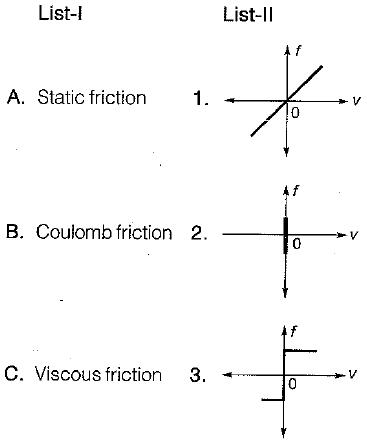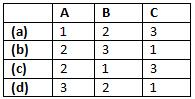Solution:
QUESTION: 5

Assertion (A): D’Alembert’s principle states that “for any- body, the algebraic sum of externally applied forces and the forces resisting motion in any given direction is zero.
”Reason (R): D’Alembert principle is used in writing the equation of motion of mechanical systems.

Solution:

Both assertion and reason are true. However, the correct reason is that all the forces in the direction of reference direction are considered as positive and the forces opposite to the reference direction are taken as negative due to which the algebraic sum of forces is zero.

QUESTION: 6

Assertion (A): When two time constant elements are cascaded interactively, the overall transfer function of such an arrangement is the product of two individual transfer functions.
Reason (R): There is loading effect present in such an arrangement.

Solution:

When two time constant elements are cascaded non-interactively, then the overall transfer function is the product of two individual TFs.
Hence, assertion is false.

QUESTION: 7

Assertion (A): An a.c. servomotor used in control system applications must have high starting torque and low inertia.
Reason (R): A large value of rotor diameter and small axial length gives a higher value of starting torque and a low value of inertia.

Solution:

Reason is a false statement because small diameter and large axial length will give a lower value of inertia of motor.

QUESTION: 8

Assertion (A): When the rotor of the synchro is inclined along any one of the stator axis completely, then this position is called electrical zero position.
Reason (R): When the rotor of the synchro is inclined along any one of the stator axis completely, then maximum voltage will be induced in that winding and almost zero voltage will be induced in the other two windings.

Solution:
QUESTION: 9

Assertion (A): Stepper motors can be used for speed control.
Reason (R): The motor speed is proportional to the rate of command pulses.

Solution:

Stepper motors can be used for speed control because the motor speed is proportional to the rate of command pulses.

QUESTION: 10

The stator of the synchros is made of

Solution:
QUESTION: 11

A synchro is used to

Solution:
QUESTION: 12

A synchro transmitter-receiver unit is a

Solution:
QUESTION: 13

The transfer function of a tachometer is of the form

Solution:
QUESTION: 14

In force-current analogy stiffness constant K is analogous to

Solution: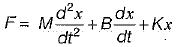and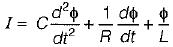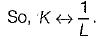QUESTION: 15

In liquid level systems, the units of capacitance of a tank is

Solution:

If q = flow; A = Area of cross-section and h = height of water in tank.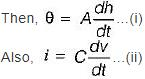On comparing equations (i) and (ii), we have C (Farad) = A = m2